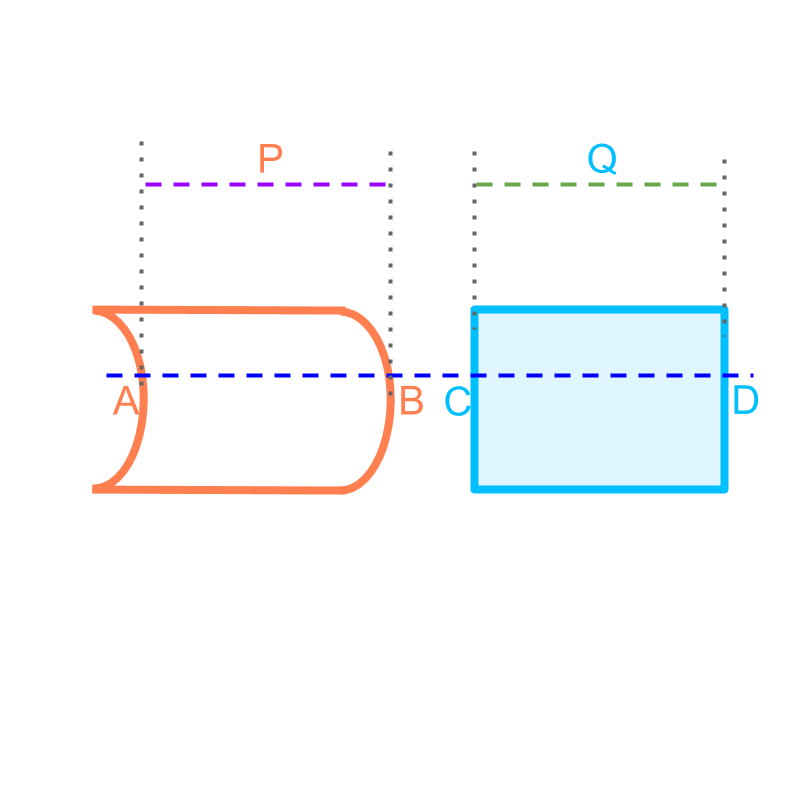maths > mensuration-high

Cavalieri's Principle (2D)

what you'll learn...

Overview

Cavalieri's Principle in 2D : For a given two shapes on a plane, a line intersecting the shapes is considered. The length of the intersecting line segments $\overline{AB}$$\overline{A B}$ and $\overline{CD}$$\overline{C D}$ are considered.If the length of the intersecting line-segments are equal for all parallel lines to the intersecting line, then the areas of the two shapes are equal.

illustrating a problemConsider the shape given in the figure. It consists of two straight lines and two curved lines. The objective is to find the area of the figure. Approximate area can be computed by superimposing unit-squares and counting them. Accurate area can be computed by some equivalence. This problem is used to illustrate and understand Cavalieri's principle.

working out equivalenceConsider finding area of the shape given in the figure. The shape is given in orange. Another shape, a rectangle, is considered. The rectangle is shown in blue.

The two figures are aligned and a line intersecting them is drawn. The point of intersections are shown as $A$$A$, $B$$B$, $C$$C$, and $D$$D$.

It is noted that the length of $\overline{AB}$$\overline{A B}$ equals the length of $\overline{CD}$$\overline{C D}$ for any position along vertical direction. These two are shown as line segments $P$$P$ and $Q$$Q$.

As per Cavalieri's principle, if the length of the line-segments are equal for any line parallel to the one shown, then the area of the two shapes are equal.

This implies that the area of the shape equals the area of the rectangle.

summary

Cavalieri's Principle in 2D : For a given two shapes on a plane, a line intersecting the shapes is considered. The length of the intersecting line segments $\overline{AB}$$\overline{A B}$ and $\overline{CD}$$\overline{C D}$ are considered.If the length of the intersecting line-segments are equal for all parallel lines to the intersecting line, then the areas of the two shapes are equal.

Outline